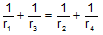Home Geometry Problems Tangential Quadrilateral     by Antonio Gutierrez
 Tangential or Circumscribed Quadrilateral: Diagonal, Inradii Theorem. The figure shows a tangential or circumscribed quadrilateral ABCD. The diagonals AC and BD meet at E. If r1, r2, r3, and r4, are the inradii of triangles AEB, BEC, CED and AED, respectively, prove that. Post a comment.Recent Additions